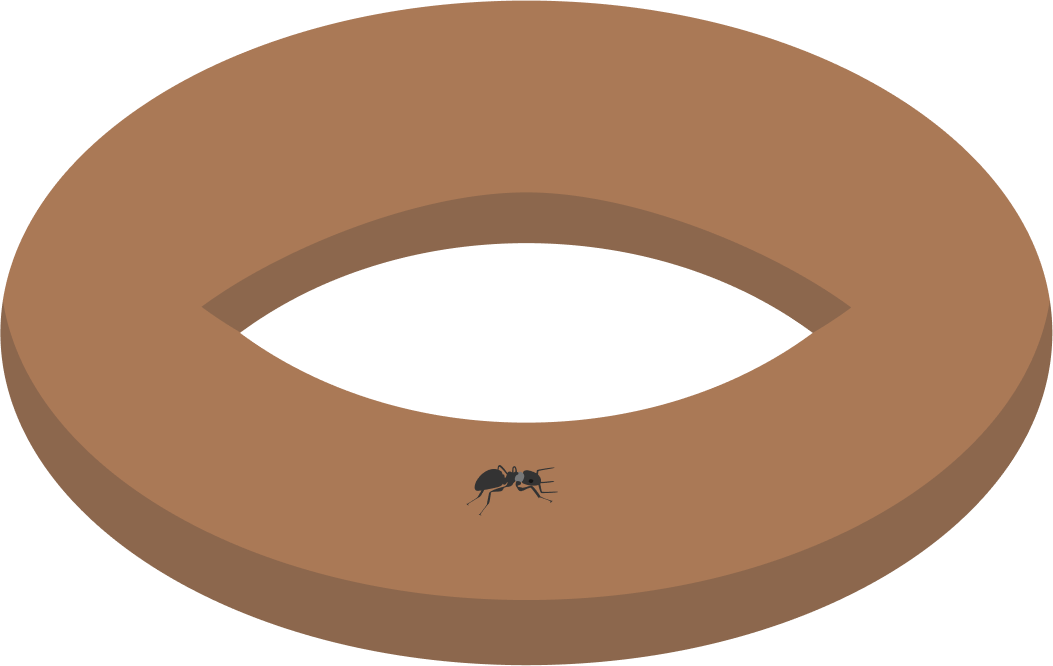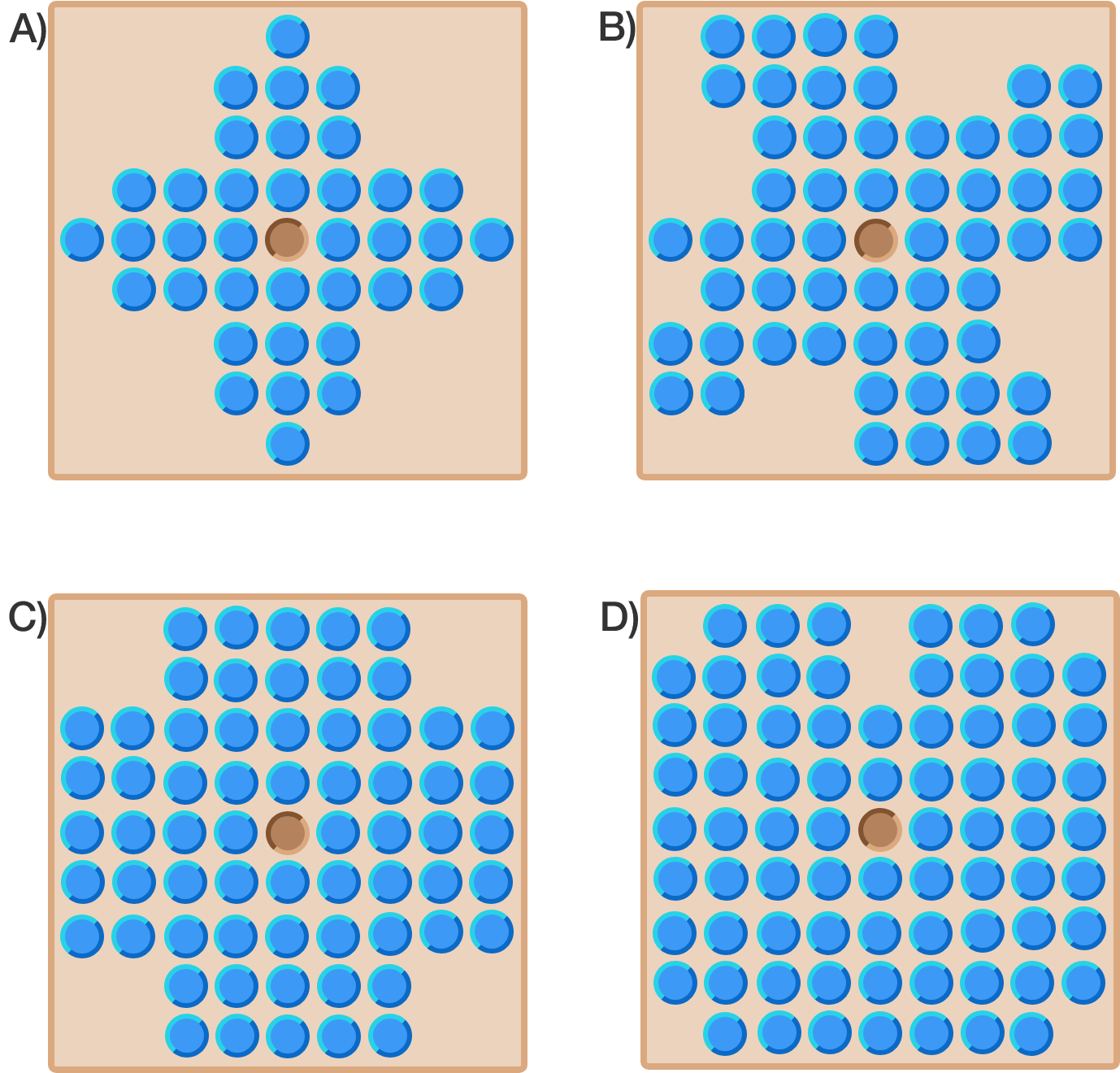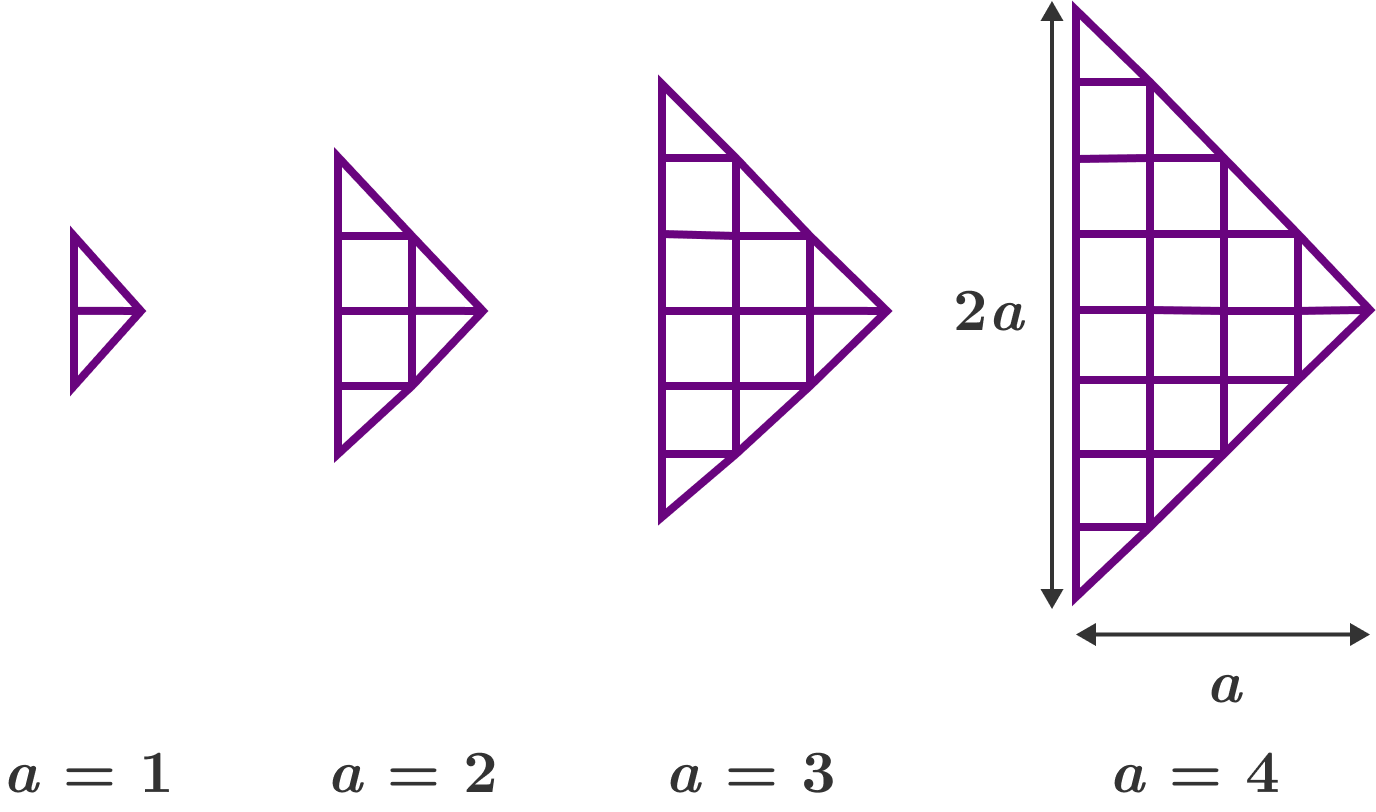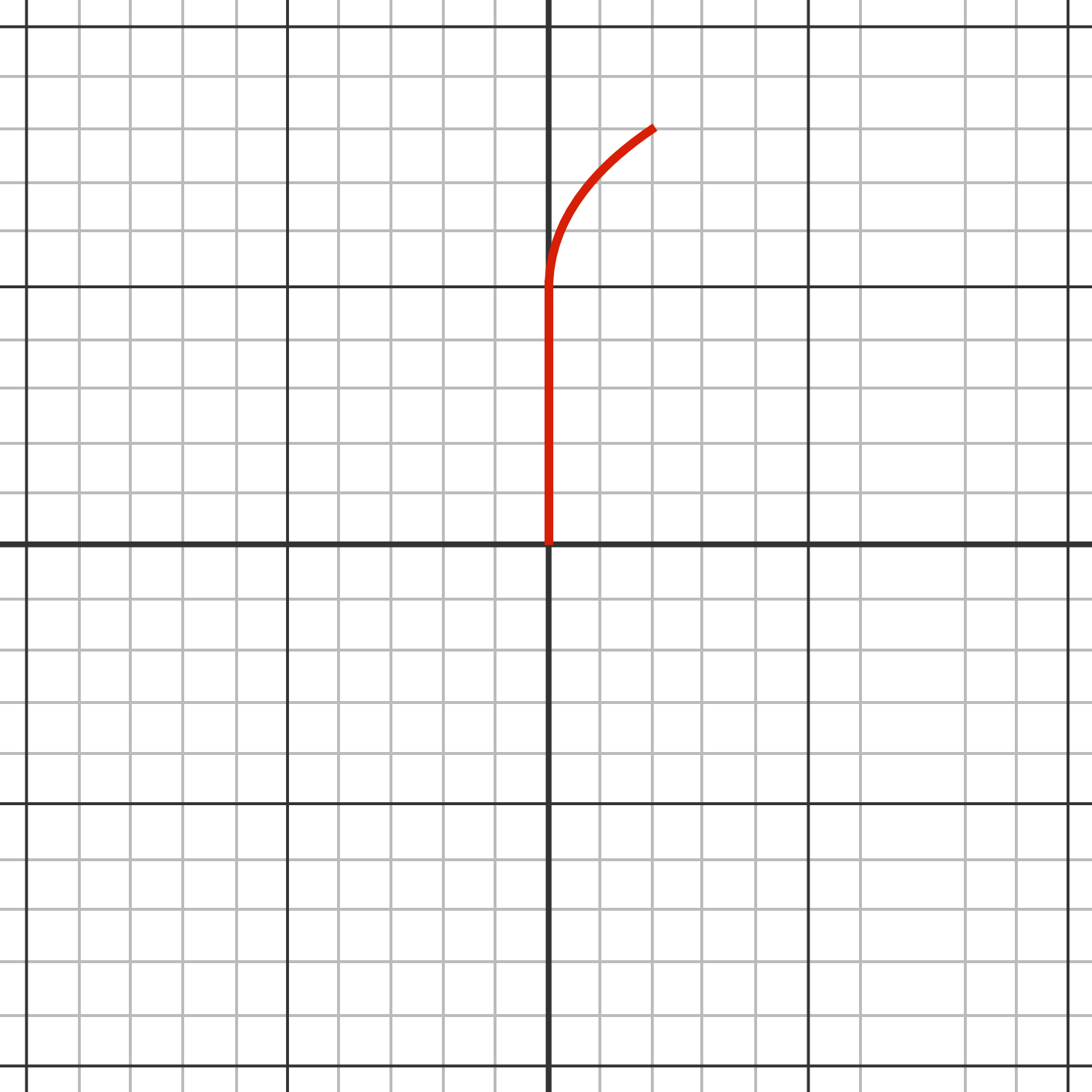# Problems of the Week

Contribute a problem

An ant crawls forward on a donut without turning.

If it crawls indefinitely like this, will it always return to its starting position at some point in time?Note: Treat the donut as a perfectly smooth torus and the ant as a point. The ant can start at any point on the donut, and can choose any starting direction.

In peg solitaire, a peg may jump over an adjacent peg vertically or horizontally into an empty hole, and the peg that was jumped over is removed. The goal of the game is to have one peg left over at the very end of the game.

When starting a game with the center peg removed, in which board can the game end with one last peg standing in the center of the board?$f(x)=x^3-3x$

Find the closed form of $f^n(x),$ and then find the number of distinct real solutions of $f^{16}(x)=2.$

Note: $f^n(x)$ is the $n^\text{th}$ composition of $f,$ that is, $f^n(x) = \underbrace{f \circ f \circ \cdots \circ f}_{n \text{ times}}(x).$

Bonus: If $g(x)=\sqrt{2+x},$ find the closed form of $g^n(x).$

A right arrow grid with base length $2a$ is defined to be an $a \times a$ grid cut into 2 halves diagonally, and then put together as illustrated.

What is the total number of quadrilaterals in a right arrow grid with $a=30?$An ant starts at the center of the image. The ant only has enough energy to walk for 10 meters (1 meter = 1 grid) on one of the highways (directly north, east, south, and west) before stopping.

However, if it goes off the highway, more energy is needed for the same distance. That is, the further the ant deviates, the greater the energy consumption, as represented by the factor $1+0.4d$, where $d$ is the distance in meters to the nearest highway. For example, if the ant is 1 meter away from the nearest highway, it uses 1.4 times the energy on the highway to walk 1 meter.

What is the area (in square meters) of the set of points the ant can reach before getting tired and stopping?The above is one possible path (in red). The ant walks 5 meters north and then curves off towards the right.

×